Next: Alternating Current Circuits Up: Direct Current Circuits Previous: Determination of Thevenin and

# Problems

1. Find the current in each resister in the circuit shown below.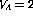V,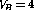V,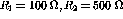and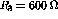. Hint: writing down the loop-current equations in terms of the symbols will give you most of the marks.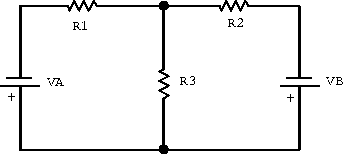2. Determine the Thevenin equivalent circuit of the circuit shown below. Hint: determine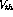and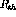.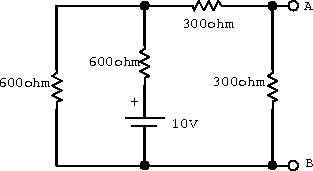3. Consider the following circuit: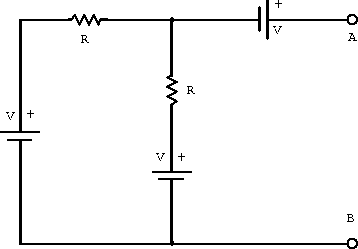1. What is the Thevenin equivalent voltage?
2. What is the Thevenin equivalent resistance?
3. For a variable load resistance placed externally between the terminals A and B, plot the current through the load as a function of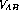. Label the intercepts on both axes.
4. Sketch the current through a load resistance as a function offor the circuit shown below. Label both intercepts and the slope.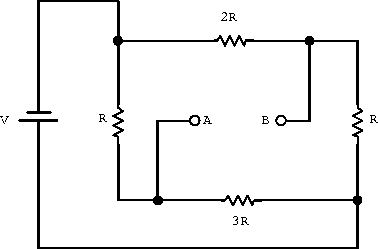5. Find the voltage,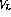, across the 3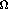load resistor for the circuit below by replacing the remaining circuit by its Thevenin equivalent. Hint: You can check your answer by direct analysis of the entire circuit.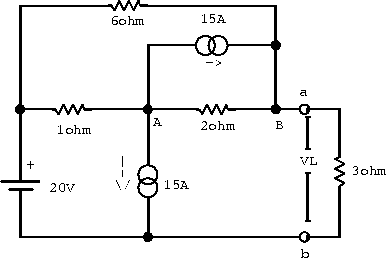Doug Gingrich
Tue Jul 13 16:55:15 EDT 1999